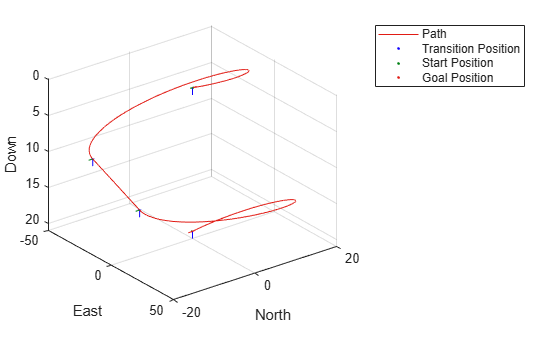# uavDubinsPathSegment

Dubins path segment connecting two poses of UAV

## Description

The `uavDubinsPathSegment` object holds information for a Dubins path segment that connects start and goal poses of a UAV as a sequence of motions in the north-east-down coordinate system.

The motion options are:

• Straight

• Left turn (counterclockwise)

• Right turn (clockwise)

• Helix left turn (counterclockwise)

• Helix right turn (clockwise)

• No motion

The turn direction is defined as viewed from the top of the UAV. Helical motions are used to ascend or descend.

## Creation

### Syntax

``pathSegObj = connect(connectionObj,start,goal)``
``pathSegObj = uavDubinsPathSegment(connectionObj,start,goal)``
``pathSegObj = uavDubinsPathSegment(connectionObj,start,goal,motionTypes)``
``pathSegObj = uavDubinsPathSegment(start,goal,flightPathAngle,airSpeed,minTurningRadius,helixRadius,motionTypes,motionLengths)``

### Description

To generate a `uavDubinsPathSegment` object, use the `connect` function with a `uavDubinsConnection` object:

````pathSegObj = connect(connectionObj,start,goal)` connects the start and goal poses using the specified `uavDubinsConnection` object. The `start` and `goal` inputs set the value of the properties StartPose and GoalPose, respectively.```

To specifically define a path segment:

````pathSegObj = uavDubinsPathSegment(connectionObj,start,goal)` creates a Dubins path segment to connect start and goal poses of a UAV. The `uavDubinsConnection` object provides the minimum turning radius and flight path angle. It internally computes the optimal path and assigns it to `pathSegObj`.```

example

````pathSegObj = uavDubinsPathSegment(connectionObj,start,goal,motionTypes)` creates a Dubins path segment to connect start and goal poses of a UAV with the given `motionTypes`. The `motionTypes` input sets the value of the MotionTypes property.```
````pathSegObj = uavDubinsPathSegment(start,goal,flightPathAngle,airSpeed,minTurningRadius,helixRadius,motionTypes,motionLengths)` creates a Dubins path segment to connect start and goal poses of a UAV by explicitly specifying all the parameters. The input values are set to their corresponding properties in the object.```

## Properties

expand all

Initial pose of the UAV at the start of the path segment, specified as a four-element numeric vector [x, y, z, headingAngle].

Data Types: `double`

Goal pose of the UAV at the end of the path segment, specified as a four-element numeric vector [x, y, z, headingAngle].

Data Types: `double`

Minimum turning radius of the UAV, specified as a positive numeric scalar in meters. This value corresponds to the radius of the circle at the maximum roll angle and a constant airspeed of the UAV.

Data Types: `double`

Helical path radius of the UAV, specified as a positive numeric scalar in meters.

Data Types: `double`

Flight path angle of the UAV to reach the goal altitude, specified as a positive numeric scalar in radians.

Data Types: `double`

Airspeed of the UAV, specified as a positive numeric scalar in m/s.

Data Types: `double`

Length of each motion in the path segment, specified as a four-element numeric vector in meters. Each motion length corresponds to a motion type specified in the MotionTypes property.

Data Types: `double`

Type of each motion in the path segment, specified as a three-element string cell array.

Motion TypeDescription
`"S"`

Straight

`"L"`

Left turn (counterclockwise)

`"R"`

Right turn (clockwise)

`"Hl"`

Helix left turn (counterclockwise)

`"Hr"`

Helix right turn (clockwise)

`"N"`

No motion

Note

The no motion segment `"N"` is used as a filler at the end when only three path segments are needed.

Each motion type corresponds to a motion length specified in the MotionLengths property.

For UAV Dubins connections, the available path types are: ```{'LSLN'} {'LSRN'} {'RSLN'} {'RSRN'} {'RLRN'} {'LRLN'} {'HlLSL'} {'HlLSR'} {'HrRSL'} {'HrRSR'} {'HrRLR'} {'HlLRL'} {'LSLHl'} {'LSRHr'} {'RSLHl'} {'RSRHr'} {'RLRHr'} {'LRLHl'} {'LRSL'} {'LRSR'} {'LRLR'} {'RLSR'} {'RLRL'} {'RLSL'} {'LSRL'} {'RSRL'} {'LSLR'} {'RSLR'}```.

Example: `{'L','R','L','N'}`

Data Types: `cell`

Length of the path segment or the flight path, specified as a positive numeric scalar in meters. This length is the sum of the elements in the MotionLengths vector.

Data Types: `double`

## Object Functions

 `interpolate` Interpolate poses along UAV Dubins path segment `show` Visualize UAV Dubins path segment

## Examples

collapse all

This example shows how to calculate a UAV Dubins path segment and connect poses using the `uavDubinsConnection` object for a specified motion type.

Create a `uavDubinsConnection` object.

`connectionObj = uavDubinsConnection;`

Define start and goal poses as [`x`, `y`, `z`, `headingAngle`] vectors.

```startPose = [0 0 0 0]; % [meters, meters, meters, radians] goalPose = [0 0 20 pi];```

Calculate a valid path segment and connect the poses for a specified motion type.

`pathSegObj = uavDubinsPathSegment(connectionObj,startPose,goalPose,{'L','S','L','N'});`

Show the generated path.

`show(pathSegObj)`Verify the motion type of the returned path segment.

`fprintf('Motion Type: %s\n',strjoin(pathSegObj.MotionTypes));`
```Motion Type: L S L N ```

## Version History

Introduced in R2019b